Home | | Maths 6th Std | Exercise 4.2

# Exercise 4.2

6th Maths : Term 3 Unit 4 : Symmetry : Exercise 4.2 : Miscellaneous Practice Problems, Challenge Problems, Text Book Back Exercises Questions with Answers, Solution

Exercise 4.2

Miscellaneous Practice Problems

1. Draw and answer the following.

i) A triangle which has no line of symmetryScalene triangle

ii) A triangle which has only one line of symmetryIsosceles triangle

iii) A triangle which has three lines of symmetryEquilateral triangle

2. Find the alphabets in the box which havei) No line of symmetry

ii) Rotational symmetry

Answer: I, O, N, X, S, H, Z

iii) Reflection symmetry

Answer: A, M, E, D, I, K, O, X, H, U, V, W

iv) Reflection and rotational symmetry

3. For the following pictures, find the number of lines of symmetry and also find the order of rotation.4. The three digit number 101 has rotational and reflection symmetry. Give five more examples of three digit numbers which have both rotational and reflection symmetry.

Answer: 181, 111, 808, 818, 888

5. Translate the given pattern and complete the design in rectangular strip?Challenge Problems

6. Shade one square so that it possessesi) One line of symmetryii) Rotational symmetry of order 2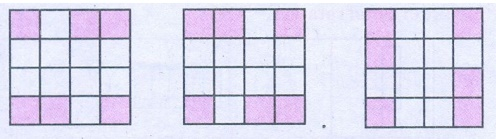7. Join six identical squares so that atleast one side of a square fits exactly with any other side of the square and have reflection symmetry (any three ways).8. Draw the following :

i) A figure which has reflection symmetry but no rotational symmetry.

ii) A figure which has rotational symmetry but no reflection symmetry.

iii) A figure which has both reflection and rotational symmetry.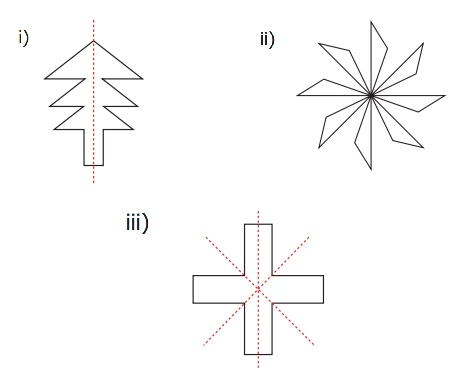9. Find the line of symmetry and the order of rotational symmetry of the given regular polygons and complete the following table and answer the questions given below.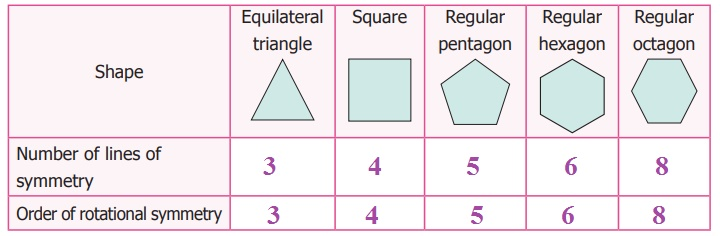i) A regular polygon of 10 sides will have 10 lines of symmetry.

ii) If a regular polygon has 10 lines of symmetry, then its order of rotational symmetry is 10.

iii) A regular polygon of 'n' sides has n lines of symmetry and the order of rotational symmetry is n.

10. Colour the boxes in such a way that it posseses translation symmetry.Exercise 4.2

1. i) Scalene triangle ii) Isosceles triangle iii) Equilateral triangle

2. i) P, N, S, Z ii) I, O, N, X, S, H, Z iii) A, M, E, D, I, K, O, X, H, U, V, W iv) I, O, X, H

3. i) 0, 2 ii) 1, 0 iii) 2, 2 iv) 8, 8 v) 1, 0

4. |8|, |||, 808, 8l8, 888

5.6.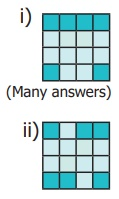7.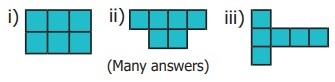8.9. i) 10 ii) 10 iii) n, n

10.Tags : Questions with Answers, Solution | Symmetry | Term 3 Chapter 4 | 6th Maths , 6th Maths : Term 3 Unit 4 : Symmetry
Study Material, Lecturing Notes, Assignment, Reference, Wiki description explanation, brief detail
6th Maths : Term 3 Unit 4 : Symmetry : Exercise 4.2 | Questions with Answers, Solution | Symmetry | Term 3 Chapter 4 | 6th Maths